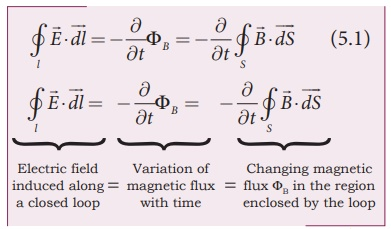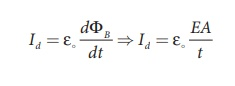Home | | Physics 12th Std | Displacement current and MaxwellŌĆÖs correction to Ampere's circuital law

# Displacement current and MaxwellŌĆÖs correction to Ampere's circuital law

Physics : Electromagnetic Waves: Displacement current and MaxwellŌĆÖs correction to Ampere's circuital law

Displacement current and MaxwellŌĆÖs correction to Ampere's circuital law

In unit 4, we studied FaradayŌĆÖs law of electromagnetic induction which states that the change in magnetic field produces an electric field. Mathematicallywhere ╬” is the magnetic flux and Ōłé/Ōłét is the partial derivative with respect to time. Equation (5.1) means that the electric fieldis induced along a closed loop by the changing magnetic flux ╬”B in the region encircled by the loop. Now the question asked by James Clerk Maxwell is ŌĆśIs converse of this statement true?ŌĆÖ Answer is ŌĆśyesŌĆÖ. He showed that the change in electric field also produces magnetic field which iswhere ╬”B is the electric flux. This is known as MaxwellŌĆÖs law of induction, which explains that the magnetic fieldinduced along a closed loop by the changing electric flux ╬”E in the region encircled by that loop. This in turn, explains the existence of radio waves, gamma rays, infrared rays, etc.

In order to understand how the changing electric field produces magnetic field, let us consider a situation of ŌĆścharging a parallel plate capacitorŌĆÖ shown in Figure 5.3 Assume that the medium in between the capacitor plates is a non-conducting medium.The electric current passing through the wire is the conduction current IC. This current generates magnetic field around the wire (refer Unit 3) connected across the capacitor. Therefore, when a magnetic needle is kept near the wire, deflection is observed. In order to compute the strength of magnetic field at a point, we use Ampere's circuital law (from Unit 3) which states that ŌĆśthe line integral of the magnetic fieldaround any closed loop is equal to ┬Ąo times the net current I threading through the area enclosed by the loopŌĆÖ. Ampere's law in equation form iswhere ┬Ąo is the permeability of free space.

To calculate the magnetic field at a point P near the wire as shown in Figure 5.4, let us draw an amperian loop (circular loop) which encloses the surface S1 (circular surface). Therefore, using Ampere's circuital law (equation 5.3), we getwhere Ic is the conduction current.

Suppose the same loop is enclosed by balloon shaped surface S2 as shown in Figure 5.5. This means that the boundaries of two surfaces S1 and S2 are same but shape of the enclosing surfaces are different (first surface (S1) is circular in shape and second one is balloon shaped surface (S2)). As the AmpereŌĆÖs law applied for a given closed loop does not depend on shape of the enclosing surface, the integrals will give the same answer. But by applying AmpereŌĆÖs circuital law (equation 5.3), we getThe right hand side of equation is zero because the surface S2 no where touches the wire carrying conduction current and further, there is no current in between the plates of the capacitor (there is a discontinuity). So the magnetic field at a point P is zero. Hence there is an inconsistency between equation (5.4) and equation (5.5). J. C.Maxwell resolved this inconsistency as follows:

Due to external source (battery or cell), the capacitor gets charged up because of current flowing through the capacitor. This produces an increasing electric field between the capacitor plates. So, there must be a current associated with the changing electric field in between the capacitor plates. In other words, the time varying electric flux (or time varying electric field) existing between the plates of the capacitor also produces a current known as displacement current.From GaussŌĆÖs law (refer Unit 1), the electric flux between the plates of the capacitor (Figure 5.6) iswhere A is the area of the plates of capacitor.

The change in electric flux iswhere Id is known as displacement current. The displacement current can be defined as the current which comes into play in the region in which the electric field and the electric flux are changing with time. In other words, whenever the change in electric field takes place, displacement current is produced. Maxwell modified Ampere's law aswhere I = Ic + Id which means the total current enclosed by the surface is sum of conduction current and displacement current. When a constant current is applied, displacement current Id = 0 and hence Ic = I. Between the plates, the conduction current Ic = 0 and hence Id = I.

EXAMPLE 5.1

Consider a parallel plate capacitor which is maintained at potential of 200 V. If the separation distance between the plates of the capacitor and area of the plates are 1 and 20 cm2. Calculate the displacement current for the time in ┬Ąs.

Solution

Potential difference between the plates of the capacitor, V = 200 V

The distance between the plates,

d = 1 mm = 1 ├Ś 10-3 m

Area of the plates of the capacitor,

A = 20 cm2 = 20 ├Ś 10-4 m2

Time is given in micro-second, ┬Ąs = 10-6 s

Displacement currentBut electric field, E = V/d

Therefore,= 35400 ├Ś10ŌłÆ7 = 3 .5 mA

Tags : Electromagnetic Waves , 12th Physics : Electromagnetic Waves
Study Material, Lecturing Notes, Assignment, Reference, Wiki description explanation, brief detail
12th Physics : Electromagnetic Waves : Displacement current and MaxwellŌĆÖs correction to Ampere's circuital law | Electromagnetic Waves